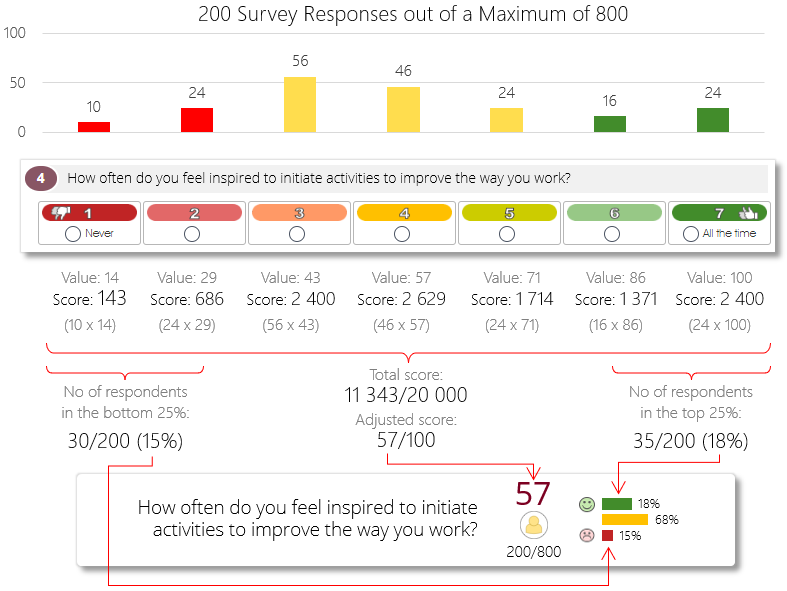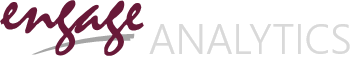Technical Guide & Help File

### Calculating Survey Question Scores

Likert survey question scores are shown as a score out of 100, and are calculated as a function of the number of respondents for each of the question’s Likert options. The calculation works like this: the number of Likert options will determine the maximum value of a response for a survey question, so a survey question with 4 Likert options will have a minimum value of 1 and a maximum value of 4, while a survey question with 7 Likert options will also have a minimum value of 1, but a maximum value of 7.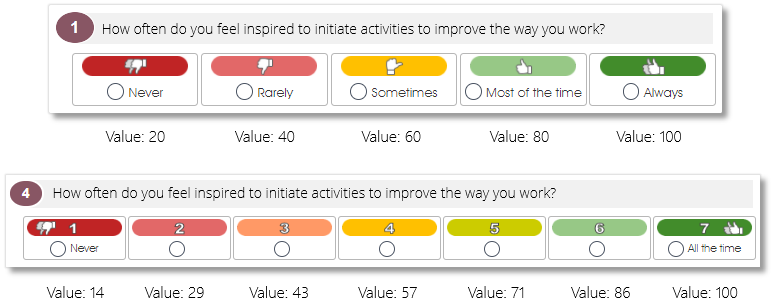The number of survey responses for each Likert option will then be multiplied by the Likert option’s value and summated to get a total score for the question, which is then recalculated so the final score can be shown out of a total of 100.

In the example below, a survey question with 4 Likert options received a total of 200 survey responses (survey sample size) out of a total of 400 potential respondents (survey population), with 100 respondents choosing the Never option (value = 1) and 100 respondents choosing the All the time option (value = 4). The number of responses for each Likert option are then multiplied with the Likert option’s value, and summated to get the final score – in this case, a final score of 500 out of a potential maximum score of 800 (the total of 200 respondents multiplied by the maximum Likert value of 4). The score is then adjusted and rounded off and shown as a value out of 100 in the analytics dashboard.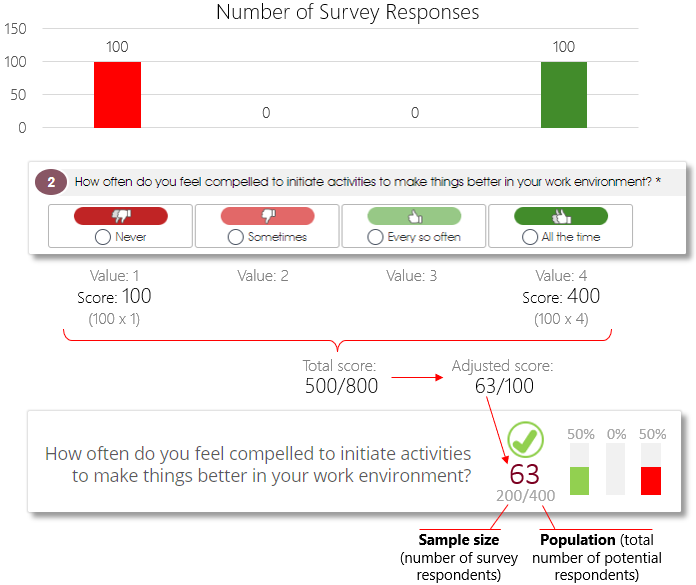The distribution of the survey responses (i.e. favorable vs. unfavorable responses) is shown via a 3-bar graph, with the number of respondents who selected the most negative Likert option (Never) shown via a red bar, and the number of respondents who selected the most positive Likert option (All the time) shown via a green bar.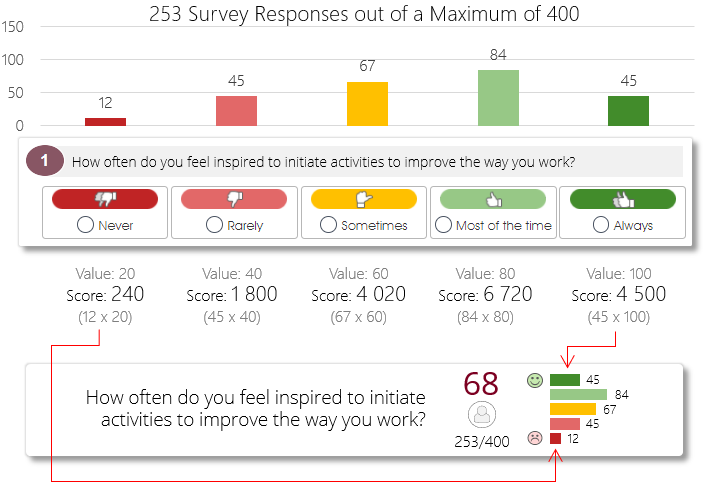Please note that the red and green bars in the distribution graph will always show the number of respondents who selected the 25% most negative and the 25% most positive Likert options as red and green bars respectively, with the remaining 50% shown as an orange bar.

The example below has a more realistic distribution of survey responses, with 34 respondents who selected the negative Never option, and 54 respondents who selected the positive All the time option. The 112 respondents who selected the middle-of-the-road Sometimes or Every so often options, are shown by means of the orange bar in the middle.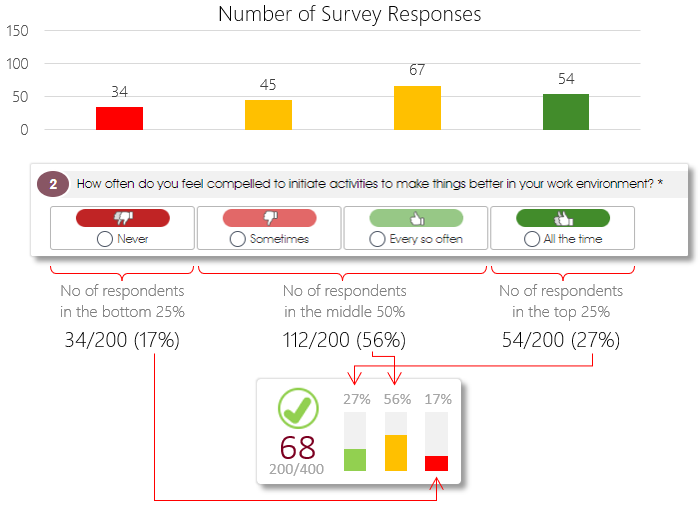Below is another example, this time with a survey question with 7 Likert options, also with a random distribution of the 200 survey responses. The number of respondents for a Likert option is again multiplied with the Likert option’s value, and summated to get the final score of 794 out of a potential maximum score of 1,400 (200 respondents multiplied by the maximum Likert value of 7), which is then adjusted and rounded off and shown as a value out of 100. The distribution graph shows the number of respondents who selected the bottom (most negative) 25% of the Likert options as a red bar, and the number of respondents who selected the top (most positive) 25% of the Likert options as a green bar.